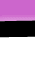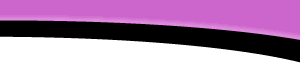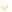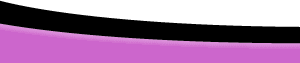HomeFAQsTutorialsResourcesHelpDreamweaverFAQ.com » Tutorials » JavaScript » Forms And Math Page 1# Forms and Math page 1

## Getting Started

This tutorial serves as an introduction to the power and limitations of JavaScript's built in Math object, a case study, convert to currency function. Remember, that part of being a good programmer is testing different scenarios and checking for desired results. Throughout this tutorial, you'll be asked to "Try it". Try various entries when possible and check those results. Doing so will give you a better understanding when reading through the explanation of the code.

Note: This tutorial is of intermediate level. You should have a basic understanding of the common terms used in JavaScript. If you are looking for a beginner level tutorial, please review JavaScript Basics- The Function before proceeding.

## Converting Numbers to Currency

JavaScript offers a number of built in Math methods (a fancy name for a function that can be attached to objects) and Math constants, take the elusive constant "PI" for instance.

`<script language="JavaScript"><!--  var pi=Math.PI;  alert(pi); } // --></script> `

Our challenge is to convert this awkward number to the currency format "\$3.14" or "£3.14" or even "€3.14". The first step is to define a function with two arguments,(1) the value to be converted (pi) and (2) the currency to be displayed:

`<script language="JavaScript"><!--function to_currency(val,currency){  //Statements that work with the arguments}// --></script>`

The programmer, at this point, should consider the "val" argument. He needs to be sure that it is indeed a number. If "val" is passed to the function from a form, it will be as a string. However, due to JavaScript's liberal policies ("loose casting" in geek terms), if you use the string in a mathematical context, it will try to convert the string to a bona fide number. There is one important exception to this rule, and that is the use of the addition (+) operator. This will be explained later.

Tip: The construction a string by adding one string to the end of another is called "concatenation". For example: alert("Today is " + new Date()) Try it!

JavaScript offers the core function "isNaN(argument)" that will inspect a value (the argument) to see if it is a number, or if it is a number that is a string. (3.14 or "3.14"). If the value is a string that can be converted to a Number, or if it is a Number, the function will return "true". One caveat: because of JavaScript's loose casting the isNaN() function will interpret an empty string as 0 (zero). Clearly an empty string can rarely be converted to currency, "\$0.00" perhaps, but for our purposes, we will consider it invalid.

Tip: To help you remember isNaN think of it like this - "Check to see if this is Not a Number".

Enter a value:

`<script language="JavaScript"><!--function to_currency(val,currency){  if(!val) {	  alert("Please provide a value");	  return;  }  if(isNaN(val)){	  alert(val+" is an invalid input");	  return;  }}// --></script>`

if(!val){alert("Please provide a value");return;} uses the "not" operator, (the exclamation point), or "if there is no val, then alert the user and terminate the function(return)"

if(isNaN(val)){alert(val+" is an invalid input"); return;} if val is not a number, then alert the user, using his invalid entry and then terminate the function. (Try placing a non-number in the box above, and click the "Check Value" button)

Remember: The concept of error checking is very important when you are dealing with user input, you need to make sure that that the input is valid.

Now that we are assured that the user input is a number, we need to convert it to the currency format "\$x.xx". We don't want the result to display as "\$.5", for example, we need it in the form "\$0.50". Since an expression such as 0.50 will be displayed as .5 in JavaScript, we are going to perform some smoke and mirrors to accomplish this.

How? We will convert the carefully triaged value into a String! But first we need to manipulate the value, using the Math.round(argument) method. This method converts its argument to the closest integer. Math.round(Math.PI) evaluates to 3, that's close, but no cigar. We need 3.14!

But if we multiply 100 by pi, then apply the the Math.round() method:
var large_pi=Math.round(100*Math.PI);

the variable "large_pi" evaluates to 314.

In this expression:
var big_three=Math.round(100*3);
"big_three" evaluates to 300.

We're almost there. We need only to insert the decimal point in the correct place. In the case of PI, decimal belongs between the three and the one. And if you remember your basic arithmetic, to divide a number by a hundred, simply move its decimal point two places to the left. The number 314's decimal point is "implied" to be to the right of the 4, (314.0). To convert the Number 314 to a string, we can use several methods, I choose to use string concatenation. String+Number results in a String.
var funny_math="1"+2
;
"funny_math" evaluates to "12" (a String). This is the exception to the "context rule" noted above. And an empty String ("") is indeed a String. The expression: ( ""+Number) is a String. We'll break the value "314" into two parts, "3" and "14" and place a decimal point between them. We'll use String.substring(index1,index2) method and the String.length property.

```<script language="JavaScript"><!--function to_currency(val,currency){  if(!val) {	  alert("Please provide a value");	  return;  }  if(isNaN(val)){	  alert(val+" is an invalid input");	  return;  }  val=""+Math.round(100*val);   var dec_point=val.length-2;  var first_part=val.substring(0,dec_point);  var second_part=val.substring(dec_point,val.length);  var result=first_part+"."+second_part;

// "3" + decimal point + "14"  return result;}// --></script>```

## The Currency Argument

To add a currency sign to the result should be a simple matter, and it is. We can make the currency argument optional, that is: if no currency argument is passed, then no currency sign will be assigned. We could add these two lines:

`// if no currency arg, set currency to empty stringif(!currency){currency="";}// if no currency arg, result =""+ result (same thing)result=currency+result; `

Add the two lines to the script as shown below:

```<script language="JavaScript"><!--function to_currency(val,currency){  if(!val) {	  alert("Please provide a value"); return;  }  if(isNaN(val)){	  alert(val+" is an invalid input");	  return;  }  val=""+Math.round(100*val);   var dec_point=val.length-2;  var first_part=val.substring(0,dec_point);  var second_part=val.substring(dec_point,val.length);  var result=first_part+"."+second_part;
if(!currency){	  currency="";  }  result=currency+result;   return result;}// --></script>```

Try it, select a currency or not, and then press the "convert" button.Copyright © 2001-2023 DreamweaverFAQ.com All Rights Reserved.